In :
# %load ../../preconfig.py
%matplotlib inline
import matplotlib.pyplot as plt
import seaborn as sns
plt.rcParams['axes.grid'] = False

#import numpy as np
#import pandas as pd
#import itertools

import logging
logger = logging.getLogger()


# 21 Data Strutures for Disjoint Sets¶

Grouping $n$ distince elements into a collection of disjoint sets.

Often need to perform two operations in particular:

• finding the unique set that contains a given element.

• uniting two sets.

### 21.1 Disjoint-set operations¶

A disjoint-set data structure maintains a collection $\delta = {S_1, S_2, \dotsc, \S_k}$ of disjoint dynamic sets.

We identify each set by a representative, which is some member of the set.

Letting $x$ denote an object, we wish to support the following operations:

1. MAKE-SET(x): $S_x = {x} : S_x \in \delta$

2. UNION(x,y): $S_x \cup S_y : x \in S_x, y \in S_y$

3. FIND-SET(x): $S_x : x \in S_x$

analyze the running times in terms of two parameters:

• $n$, the number of MAKE-SET operations,

• $m$, the total number of all kinds of operations.

The number of UNION operations must be less than MAKE-SET's, namely, $< n$. And we also have $m \geq n$.

An application: determining the connected components of an undirected graph.

In :
plt.imshow(plt.imread('./res/find_connected.png'))

Out:
<matplotlib.image.AxesImage at 0x1092a10b8>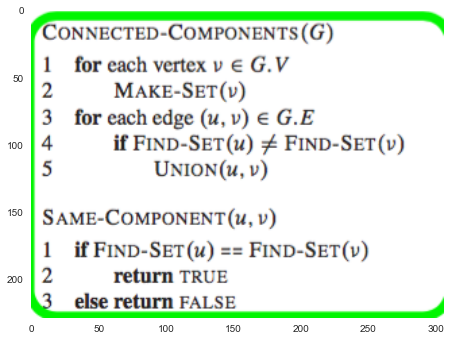In :
plt.figure(figsize=(12,8))

Out:
<matplotlib.image.AxesImage at 0x10ab5c3c8>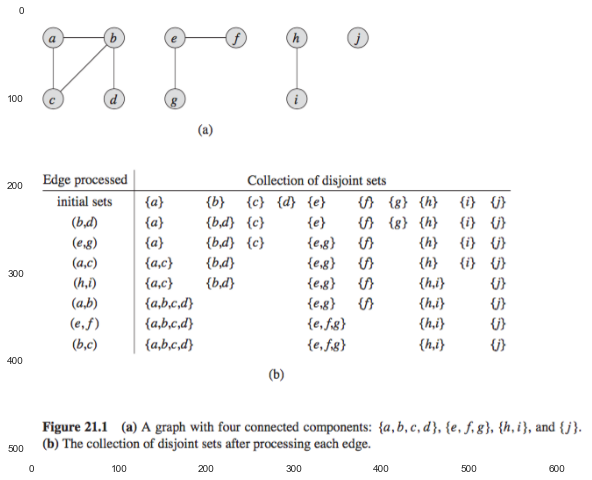In :
# Exercises


### 21.2 Linked-list representation of disjoint sets¶

1. Each set:

• tail.

2. Each set member:

• a pointer to the next object in the list.

• a pointer back to the set object.

In :
plt.figure(figsize=(15,8))

Out:
<matplotlib.image.AxesImage at 0x10b88ccf8>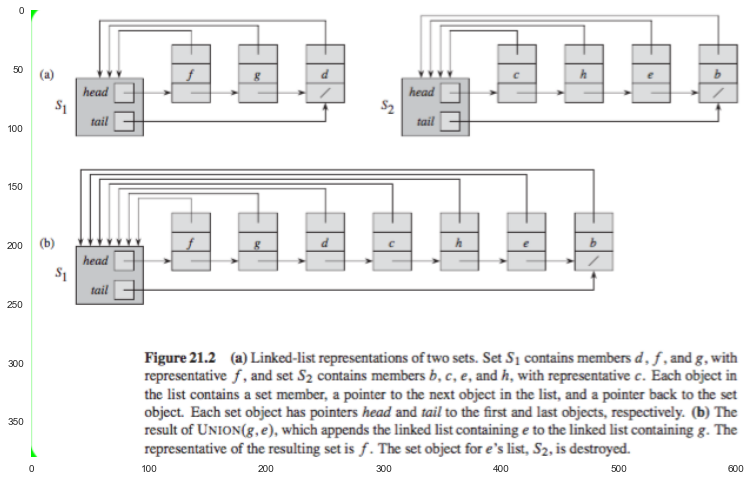MAKE-SET and FIND-SET requires $O(1)$ time.
note: FIND-SET(x), where $x$ is the pointer to the object expected.

UNION:

• randomly merge: append one to another list, and update the related pointer. $O(n)$.

• construct a sequence of $m$ operations on $n$ objects that requires $O(n^2)$ time.
• weighted-union heuristic: append the shortest list onto the longer. $\Omega(n)$

• each list need to include the length of the list.

• construct a sequence of $m$ operations on $n$ objects that requires $O(m + n \lg n)$ time.

In :
# Exercises


### 21.3 Disjoint-set forests¶

Disjoint-set forests: We represent sets by rooted trees, with each node containing one member and each tree representing one set.

#### union by rank¶

For each node, we maintain a rank, which is an upper bound on the height of the node.

UNION operation: We make the root with smaller rank point to the root with larger rank.

In :
plt.imshow(plt.imread('./res/fig21_4.png'))

Out:
<matplotlib.image.AxesImage at 0x1096afd30>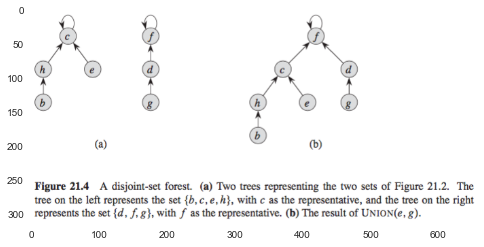#### path compression¶

FIND-SET: We make each node on the find path point directl to the root.

In :
plt.imshow(plt.imread('./res/fig21_5.png'))

Out:
<matplotlib.image.AxesImage at 0x10a5f3470>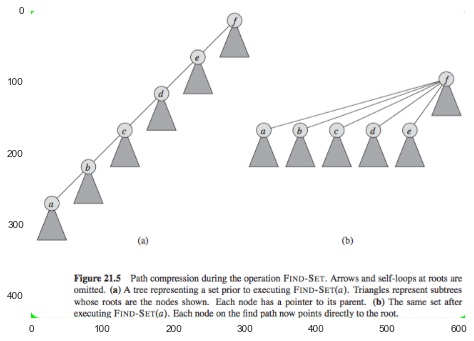In :
# Pseudocode for disjoint-set forests

<matplotlib.image.AxesImage at 0x10b053f60>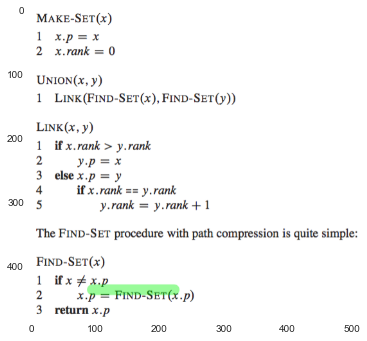When we use both union by rank and path compression, the worst-case running time is $O(m \alpha(n))$, where $\alpha(n)$ is a very slowly growing function, which we define in Section 21.4.
# Exercises